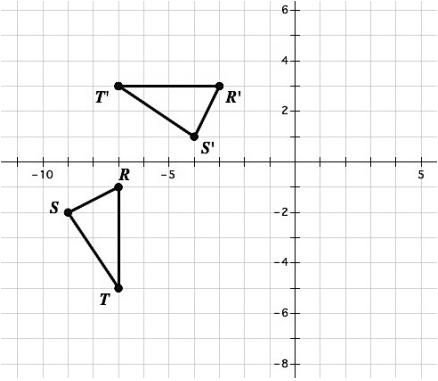Rotational Symmetry
Reflection
Reflection and Rotation
Sequence of Transformations
100

Reflect over the line

y=-x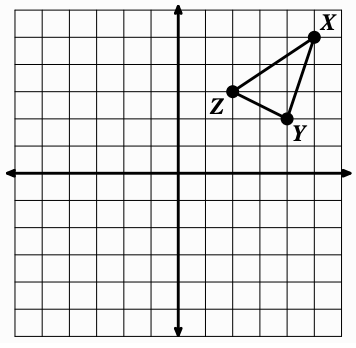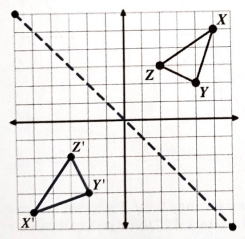200

Reflect over the y-axis.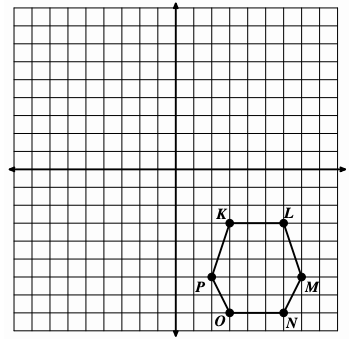200

Rotate point P 90º clockwise around point C.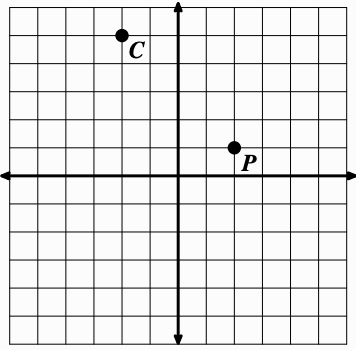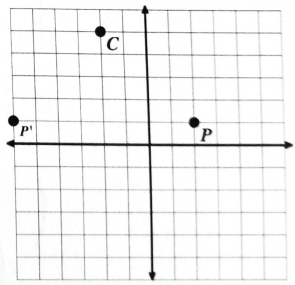300

What angles of rotational symmetry are there for a regular pentagon?

Multiples of 72° (72, 144, 216, 288, 360)

300

Reflect triangle ABC over the line:

y=x

and label the image A'B'C'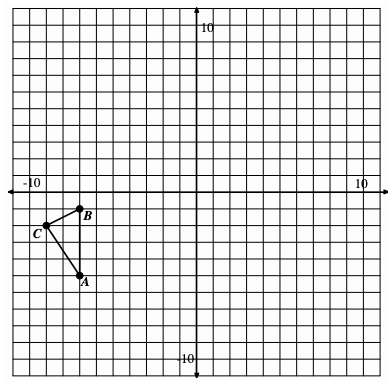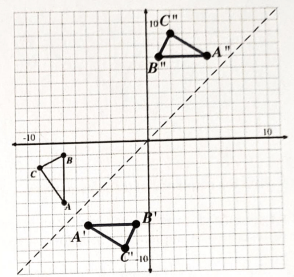300

Reflect over the y-axis and then rotate clockwise 90° around P'400

What angles of rotational symmetry are there for a regular hexagon?

Multiples of 60° (60, 120, 180, 240, 300, 360)

400

Reflect quadrilateral ABCD over the line:

y=2+x

and label the image A'B'C'D'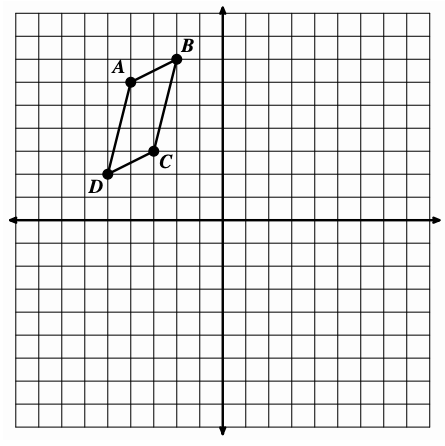400

Reflect triangle ABC over the line:

y=x

and label the image A'B'C'.

Rotate A'B'C' 180° counter-clockwise around the origin and label the image A''B''C''400

Find a sequence of transformations that will carry triangle RST onto triangle R’S’T’. Clearly describe the sequence of transformations.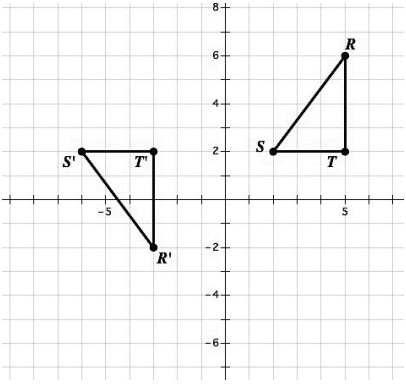Translate left 8 so S coincides with S'

Reflect across S'T' so R lands on R'

500

If a regular polygon has an angle of rotational symmetry that is 40°, how many sides does the polygon have?

9 sides

500

Reflect point P over line j.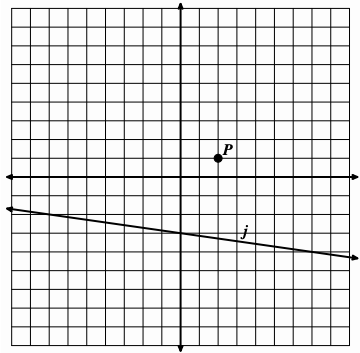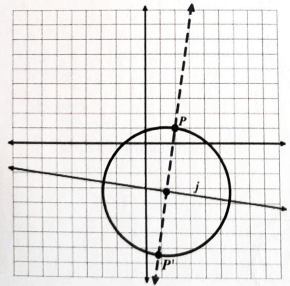500

Reflect quadrilateral ABCD over the line:

y=2+x

and label the image A'B'C'D'.

Rotate quadrilateral A'B'C'D' counter-clockwise 90° around (-2, -3) as the center of rotation and label the image A''B''C''D''.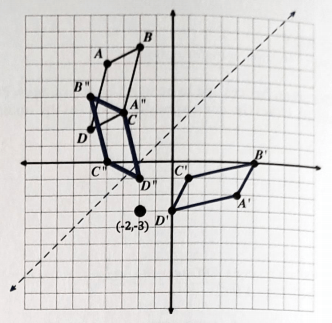500

Find a sequence of transformations that will carry triangle RST onto triangle R’S’T’. Clearly describe the sequence of transformations.